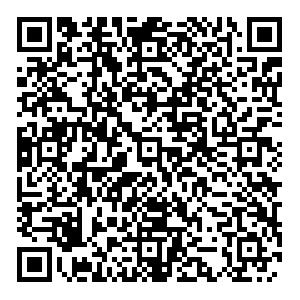# X0(2900) and its heavy quark spin partners in molecular picture• The $X_0(2900)$, recently observed by the LHCb Collaboration in the $D^-K^+$invariant mass of the $B^+\to D^+D^-K^+$process, is the first exotic candidate with four different flavors, beginning a new era for the hadron community. Under the assumption that the $X_0(2900)$is a $I(J^P)=0(0^+)$$\bar{D}^*K^*$hadronic molecule, we extracted the whole heavy-quark symmetry multiplet formed by the $\left(\bar{D},\bar{D}^*\right)$doublet and the $K^*$meson. For the bound state case, there would be two additional $I(J^P)=0(1^+)$hadronic molecules associated with the $\bar{D}K^*$and $\bar{D}^*K^*$channels, as well as one additional $I(J^P)=0(2^+)$$\bar{D}^*K^*$molecule. In the light quark limit, they are $36.66~{\rm{MeV}}$and $34.22~{\rm{MeV}}$below the $\bar{D}K^*$and $\bar{D}^*K^*$thresholds, respectively, which are unambiguously fixed by the mass position of the $X_0(2900)$. For the virtual state case, there would be one additional $I(J^P)=0(1^+)$hadronic molecule, strongly coupled to the $\bar{D}K^*$channel, and one additional $I(J^P)=0(2^+)$$\bar{D}^*K^*$molecule. Searching for these heavy quark spin partners will help shed light on the nature of the $X_0(2900)$.
••Get Citation
Mei-Wei Hu, Xue-Yi Lao, Pan Ling and Qian Wang. The X0(2900) and its heavy quark spin partners in molecular picture[J]. Chinese Physics C.
Mei-Wei Hu, Xue-Yi Lao, Pan Ling and Qian Wang. The X0(2900) and its heavy quark spin partners in molecular picture[J]. Chinese Physics C.Milestone
Article Metric

Article Views(79)
Cited by(0)
Policy on re-use
To reuse of Open Access content published by CPC, for content published under the terms of the Creative Commons Attribution 3.0 license (“CC CY”), the users don’t need to request permission to copy, distribute and display the final published version of the article and to create derivative works, subject to appropriate attribution.
###### 通讯作者: 陈斌, bchen63@163.com
• 1.

沈阳化工大学材料科学与工程学院 沈阳 110142

Title:
Email:

## X0(2900) and its heavy quark spin partners in molecular picture

###### Corresponding author: Qian Wang, qianwang@m.scnu.edu.cn
• 1. Guangdong Provincial Key Laboratory of Nuclear Science, Institute of Quantum Matter, South China Normal University, Guangzhou 510006, China
• 2. Guangdong-Hong Kong Joint Laboratory of Quantum Matter, Southern Nuclear Science Computing Center, South China Normal University, Guangzhou 510006, China
• 3. Institute of High Energy Physics, Chinese Academy of Sciences, Beijing 100049, China

Abstract: The $X_0(2900)$, recently observed by the LHCb Collaboration in the $D^-K^+$invariant mass of the $B^+\to D^+D^-K^+$process, is the first exotic candidate with four different flavors, beginning a new era for the hadron community. Under the assumption that the $X_0(2900)$is a $I(J^P)=0(0^+)$$\bar{D}^*K^*$hadronic molecule, we extracted the whole heavy-quark symmetry multiplet formed by the $\left(\bar{D},\bar{D}^*\right)$doublet and the $K^*$meson. For the bound state case, there would be two additional $I(J^P)=0(1^+)$hadronic molecules associated with the $\bar{D}K^*$and $\bar{D}^*K^*$channels, as well as one additional $I(J^P)=0(2^+)$$\bar{D}^*K^*$molecule. In the light quark limit, they are $36.66~{\rm{MeV}}$and $34.22~{\rm{MeV}}$below the $\bar{D}K^*$and $\bar{D}^*K^*$thresholds, respectively, which are unambiguously fixed by the mass position of the $X_0(2900)$. For the virtual state case, there would be one additional $I(J^P)=0(1^+)$hadronic molecule, strongly coupled to the $\bar{D}K^*$channel, and one additional $I(J^P)=0(2^+)$$\bar{D}^*K^*$molecule. Searching for these heavy quark spin partners will help shed light on the nature of the $X_0(2900)$.

### HTML关注分享DownLoad:  Full-Size Img  PowerPoint